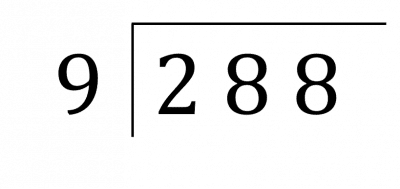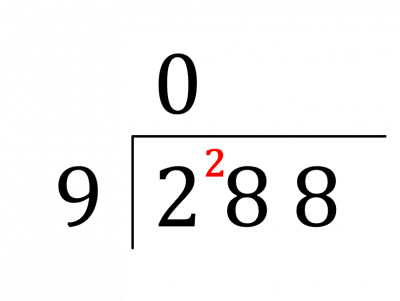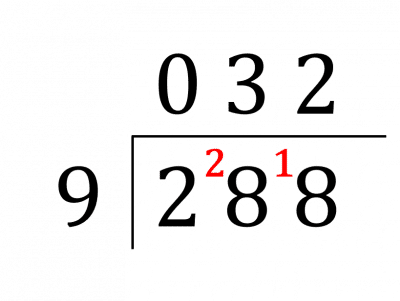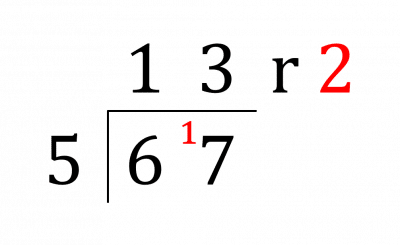# Long Division – The Bus Stop Method

GCSEKS3Level 1-3AQAEdexcelOCRWJEC

## Long Division

There are different methods that can be used for long division but the most commonly used in the UK is the Bus Stop method.

Level 1-3GCSEKS3AQAEdexcelOCRWJEC

## Bus Stop Method

This method for long division is called the Bus Stop method, since it looks like a bus stop. This method allows us to divide numbers to find integer answers or answers that have a remainder. There are a few steps that you need to follow.

Example: Calculate $288 \div 9$.

Step 1: We start by putting the division into the Bus Stop form as show below.Step 2: We see how many times $9$ goes into $2$, the first digit. The answer is $\textcolor{blue}{0}$ so the $\textcolor{red}{2}$ is carried onto the next digit to make a new number, $28$.  The zero goes at the top.Step 3: Now, we see how many times $9$ goes into $28$. We know that $3 \times 9=27$, so $9$ goes into $28$ $\textcolor{blue}{3}$ times with a remainder $\textcolor{red}{1}$, which is then carried in front of the next digit to make a new number, $18$. The three goes at the top.

Step 4: Then, we see how many times the $9$ goes into $18$, which is $\textcolor{blue}{2}$. The two then goes at the top. These two steps are combined in the image below.So,

$288 \div 9 = \textcolor{limegreen}{32}$

Note: If $9$ did not go into the number in the last step, for example if the question asked to calculate $289 \div 9$, then $9$ goes into $19$ $\textcolor{blue}{2}$ times, with a remainder $\textcolor{red}{1}$. So we would say that the answer had a remainder of $1$ and we would write

$289 \div 9 = \textcolor{limegreen}{32} \text{ r } \textcolor{red}{1}$

Level 1-3GCSEKS3AQAEdexcelOCRWJEC

## Example: Long Division with a Remainder

Calculate $67 \div 5$

[2 marks]

We use the same bus stop method as shown before, but this time we have a remainder.$67 \div 5 = \textcolor{limegreen}{13} \text{ r } \textcolor{red}{2}$

Level 1-3GCSEKS3AQAEdexcelOCRWJEC

## Long Division – The Bus Stop Method Example Questions

Here we’re going to use the bus stop method.

$14$ goes into $3$ zero times, so we write a zero above the line and a small $3$ – the remainder – in between the $3$ and the $1$. Then, we see how many times $14$ goes into $31$, writing the result above the line and the remainder just before the next digit of the dividend.

Repeat this process until you get the complete answer, which looks like,

$\;\;\;\;\;0\;2\;2\;.\;5\\14\overline{\left)3{}^31{}^35.{}^70\right.}$Gold Standard Education

Here we’re going to use the bus stop method,

$15$ goes into $2$ zero times, so we write a zero above the line and a small $2$ – the remainder – in between the $2$ and the $2$. Then, we see how many times $15$ goes into $22$, which is $1$,  writing the result above the line and the remainder, $7$,  just before the next digit of the dividend.

Repeat this process until you get the complete answer, which looks like,

$\;\;\;\;\;0\;1\;5\\15\overline{\left)2{}^22{}^75\right.}$Gold Standard Education

Here we’re going to use the bus stop method,

$160$ goes into $4$ zero times, so we write a zero above the line and a small $4$ – the remainder – in between the $4$ and the $3$. Then, we see how many times $160$ goes into $43$, which is also zero,  writing the result above the line and the remainder, $43$,  just before the next digit of the dividend.

Repeat this process until you get the complete answer, which looks like,

$\;\;\;\;\;\;\;0\;\;0\;\;2\;\;7\\160\overline{\left)4{}^43{}^{43}2{}^{112}0\right.}$Gold Standard Education

Here we’re going to use the bus stop method,

$13$ goes into $2$ zero times, so we write a zero above the line and a small $2$ – the remainder – in between the $2$ and the $9$. Then, we see how many times $13$ goes into $29$, which is $2$,  writing the result above the line and the remainder, $3$,  just before the next digit of the dividend.

Repeat this process until you get the complete answer, which looks like,

$\;\;\;\;0\;2\;3\\13\overline{\left)2{}^29{}^39\right.}$Gold Standard Education

Here we’re going to use the bus stop method,

$9$ goes into $2$ zero times, so we write a zero above the line and a small $2$ – the remainder – in between the $2$ and the $3$. Then, we see how many times $9$ goes into $23$, which is $2$, writing the result above the line and the remainder, $5$, just before the next digit of the dividend, so we find,

$\;\;\;0\;2\;6\\9\overline{\left)2{}^23{}^54\right.}\\\;\;\;\;\;\;\;\;$